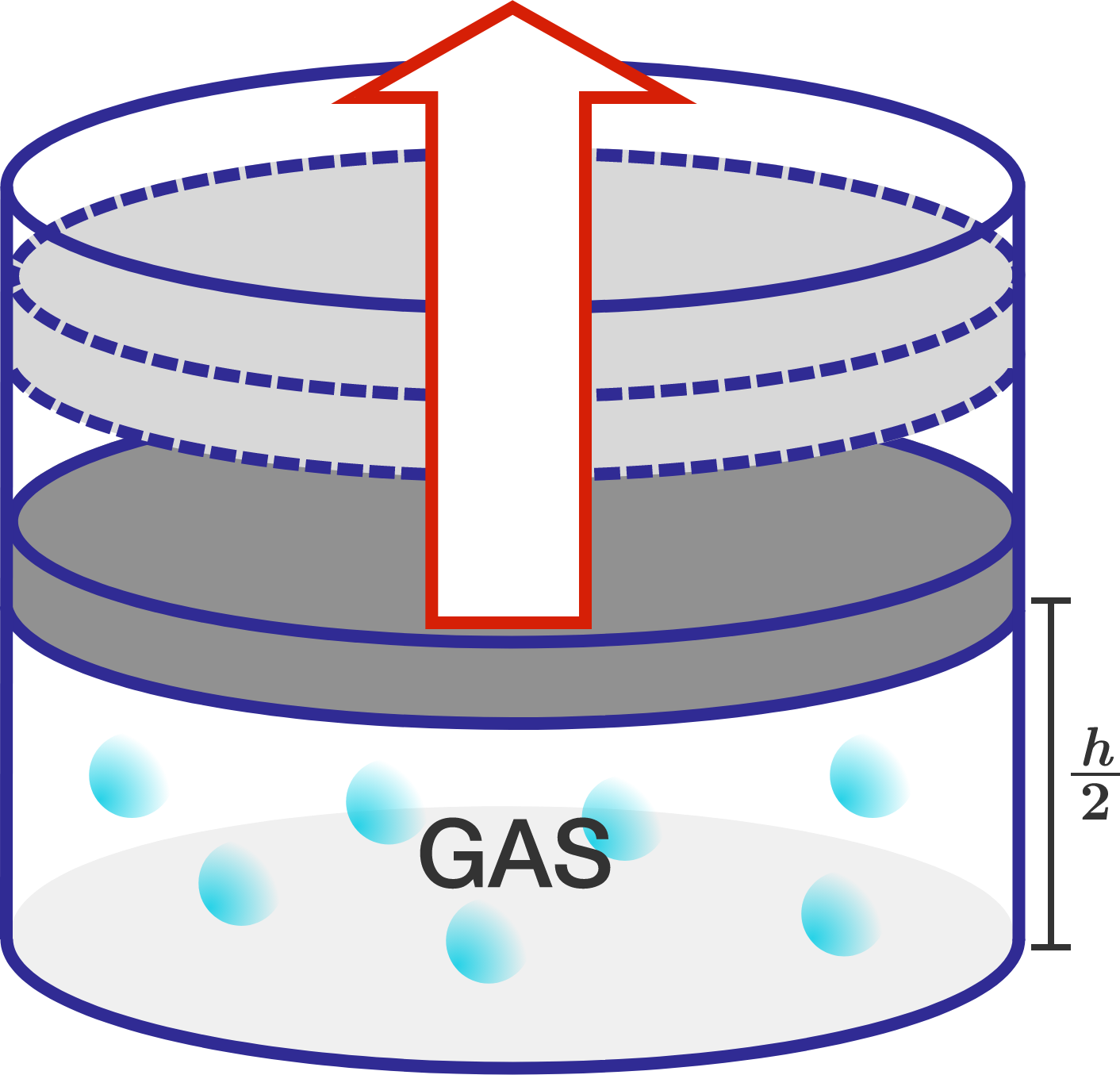# Thermodynamic PistonA cylindrical chamber of cross sectional area $\SI{4}{\centi\meter\squared}$ is filled with an ideal gas, and a piston of mass $\SI{2}{\kilo\gram}$ sits at the midpoint of the cylinder. The rest of the chamber has total mass $M.$

Now, the piston is pulled very slowly upward. If the temperature of the gas inside the chamber remains constant, find the maximum value of $M$ $($in $\text{kg})$ such that the chamber can be lifted off the ground in this way. Submit your answer to 2 decimal places.


Details and Assumptions:

• There is negligible friction in the system.
• Atmospheric pressure is $10^5 \text{ N/m}^2,$ and $g=10 \text{ m/s}^2.$
×